Related Articles

# How to Calculate Mean Squared Error in Excel?

• Last Updated : 29 Jun, 2021

Mean Squared Error is defined as the mean of the square of the difference between the actual values and the expected values.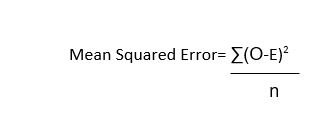Attention reader! Don’t stop learning now. If you are an Excel beginner (or an intermediate) and want to learn Excel, Geeksforgeeks brings the perfect course for you to start, Diving Into Excel

Where,

• O = Observed value,
• E = Expected Value,
• n = No. of observations

Example:

Follow the below steps to evaluate the MSE in Excel:

Step 1: Suppose we are given the data in form of expected and observed value as follows: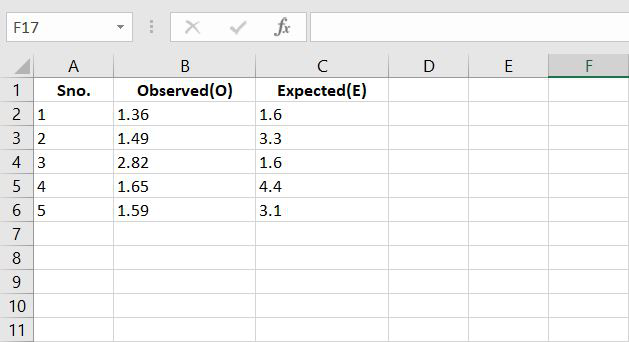Step 2: Now let’s calculate the difference between the observed and the expected value.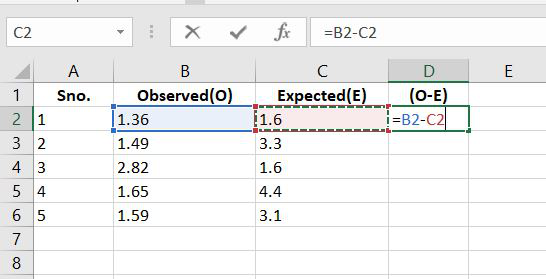Step 4: Now calculate the square of the difference.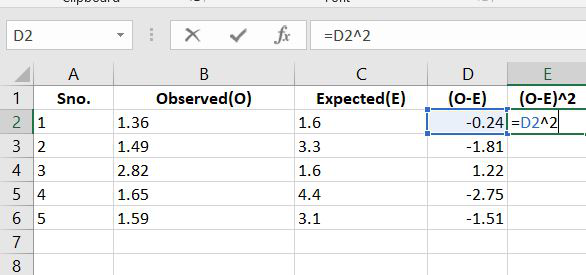Step 5:  We will now calculate the sum of the squared values.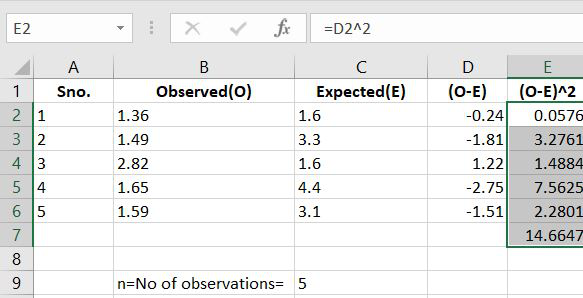Step 6: Now we have the value of the sum of squared values also we have the no. of observations i.e. 5. Now put these values in the MSE formulae and get the final result.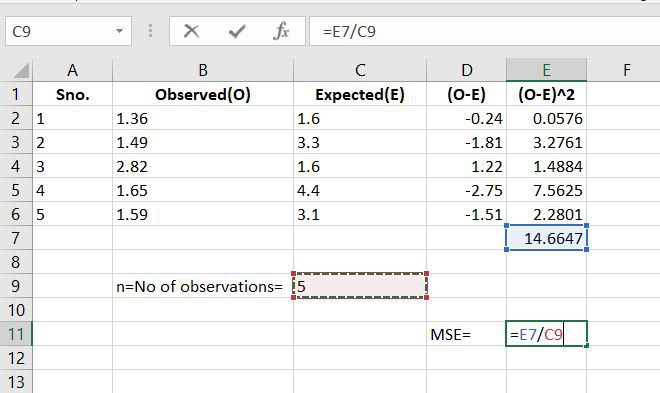Step 7: Now by putting the values in the formulae we get the Mean Squared Error for the given data set as 2.93294.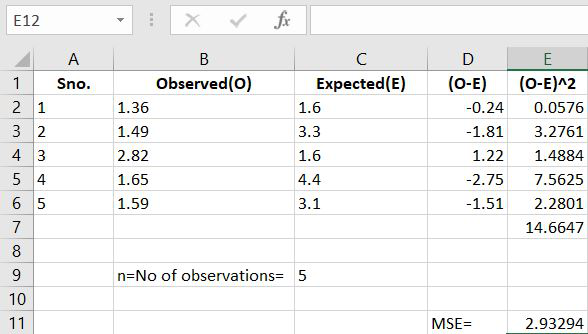My Personal Notes arrow_drop_up